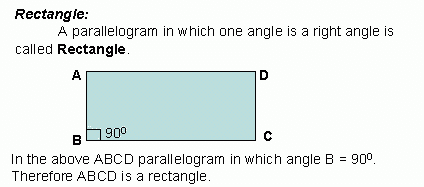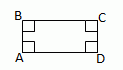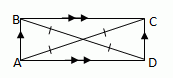Name: ___________________Date:___________________Properties of Rectangle: Opposite sides of a rectangle are equal or congruent. Opposite angles of a rectangle are equal. All its angles are right angles; or The diagonals of a rectangle bisect each other. Each diagonal divides the rectangle into two congruent triangles. The diagonals of a rectangle are equal. Both pairs of opposite sides are equal and one angle is 90Ḟ; or Both pairs of opposite sides are equal and its diagonals are equal. THEOREMS A quadrilateral is a rectangle if and only if it has four right angles.ŴA, ŴB, ŴC, ŴD are 900 A parallelogram is a rectangle, if and only if its diagonals are congruent.AC @ BD Directions: Read the above review points carefully and answer the following questions: Illustrate each of the above review points by drawing a Rectangle. Explain the different properties of a Rectangle, in your own words, with examples. Lilly measures her 10 cm x 6 cm garden plot. She found that all its edges are of angle measure 90Ḟ. What will be the shape of her garden? Give reasons. What will be the value of one of the angles of a rectangle if one side is given by 17 cm and other side by 12 cm? Give reasons. If the lengths of the rectangle is x+31 and 5x-9 what is its length. For the following rectangle is the diagonal AC=10, find the mŴDOA, mŴBOC, BO, OD, AO, OC, AC